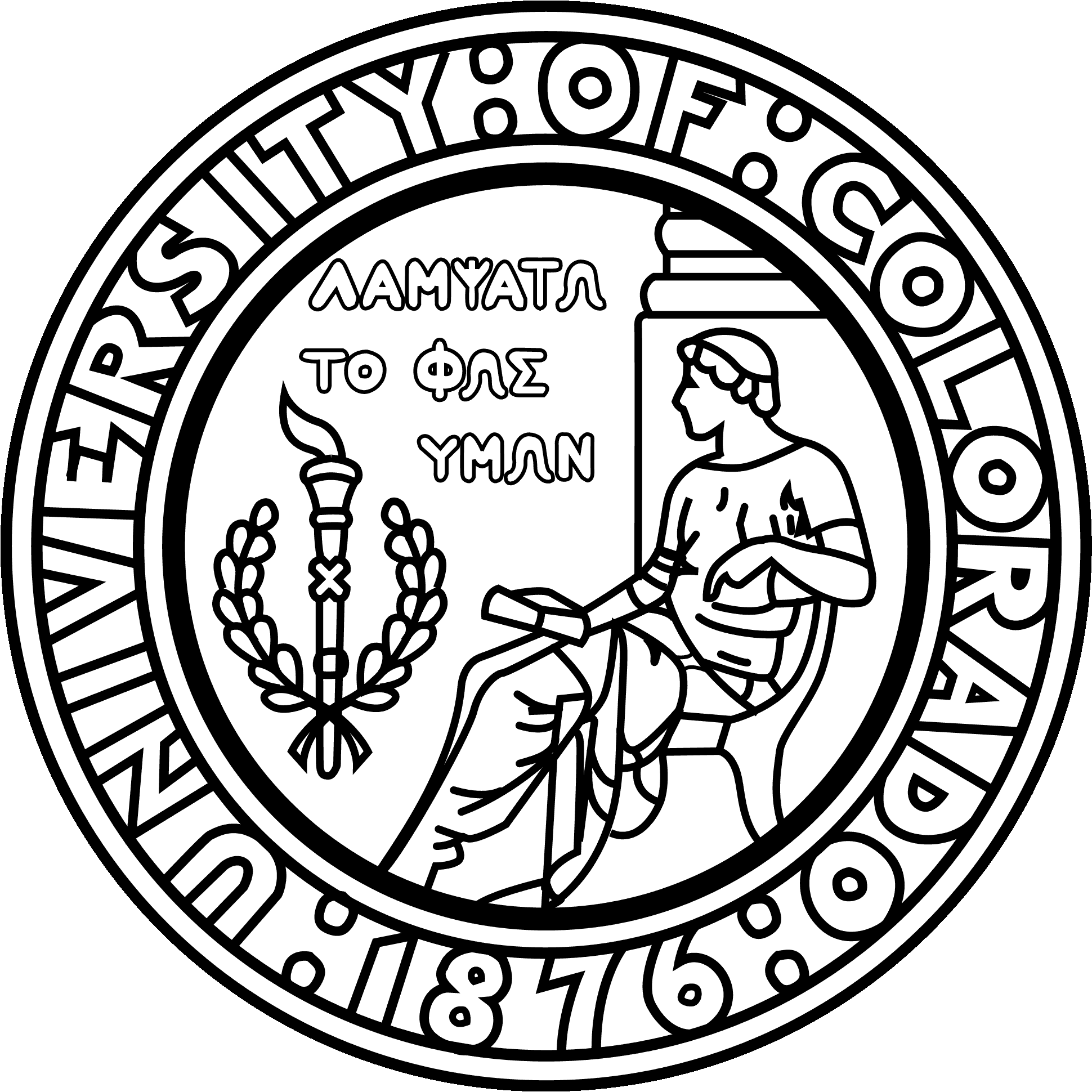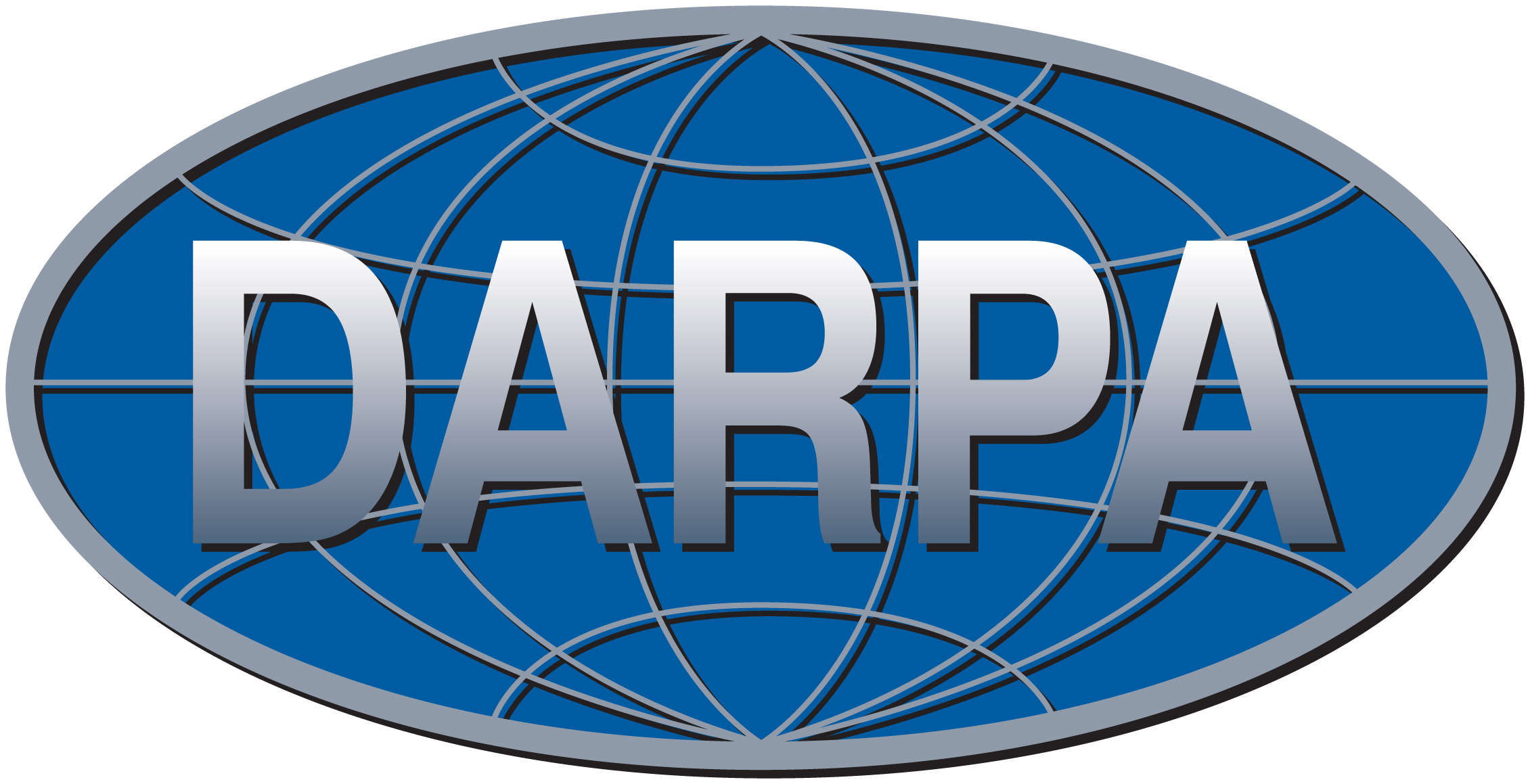CBMS/NSF Conference on Fast Direct Solvers, June 2014

Dartmouth College, June 23 - 27, 2014

 Home Lectures Codes Bibliography Support

 Lecture 1 Lecture 2 Lecture 3 Lecture 4 Lecture 5 Lecture 6 Lecture 7 Lecture 8 Lecture 9 Lecture 10

This page provides links to accessible references, and to software for the material covered in each of the lectures.

References to original works are provided in the bibliography.
• Lecture 1: Introduction to fast direct solvers for elliptic PDEs

The material on classical solution methods for solving the Laplace equation can be found in most standard references on mathematical physics. Descriptions of multipole expansions can be found in most works describing the Fast Multipole Method, including the dissertation of Leslie Greengard, a short course on FMMs by Rick Beatson and Leslie Greengard, and a brief encyclopedia entry.

The codes used to generate various solutions to Laplace equation are here.

• Lecture 2: The classical Fast Multipole Method

The following encyclopedia entry provides more details on the material in the lecture, and follows the same notation.

Detailed descriptions of the FMM are given in, e.g, the dissertation of Leslie Greengard, a short course on FMMs by Rick Beatson and Leslie Greengard, a tutorial by Liu and Nishimura, and a variety of books, including this by Chew, Jin, Michielssen, and Son, and this by Yijun Liu.

A tutorial style Matlab implementation of the FMM can be downloaded here. These codes were used to produce many of the graphical illustrations in the lecture.

For serious implementations of the FMM, check, e.g., here or here.

A general collection of references on the FMM can be found here.

• Lecture 3: The Interpolative Decomposition (ID)

For a very cursory description, see Section 3.2 of this survey. A full technical paper is here.

Codes for computing IDs can be found here. Note that there is no entirely satisfactory way of computing partial IDs (or any other partial decomposition) in Matlab.

The figures that compare the errors incurred by the SVD, the ID, multipole expansions, and RSVD were generated by the following files: Laplace, Helmholtz.

• Lecture 4: Brief introduction to structured matrix algebra

An introduction to structured matrix algebra following the notation in the lecture can be found here (extract from a draft of the monograph manuscript).

Sandbox codes for playing with S-matrices here.

• Lecture 5: Randomized methods for low-rank approximation

The original paper we published on these methods is this 2006 tech report. The report was (after two rejections...) eventually published here in ACHA.

An accessible survey. It is very long, but the introduction can be read by itself as a quick introduction. A briefer introduction is given in this 2011 PNAS paper.

Sandbox codes for the "rsvd" here.

• Lecture 6: Fast direct solvers for sparse matrices

Code used to test compressibility of Schur complements here.

2009 SIMAX paper by Ming Gu, J. Xia, S. Chandrasekaran, M. Gu, and X.S. Li here. This has later been followed up by several papers by Jianlin Xia, Maarten Van de Hoop, and others at Purdue U., see, e.g. here.

A slightly outdated, but (I think!) accessible description of the ideas can be found in this 2009 paper from our group (developed independently from the work by Xia, Gu, Chandrasekaran and Li).

Work on so called H-LU methods which use H-matrix algebra to accelerate the nested dissection method by Lars Grasedyck, Sabine Le Borne, and Ronald Kriemann can be found in this 2007 paper.

Related work by Philip Schmitz and Lexing Ying is here, and by Ken Ho and Lexing Ying on techniques for more easily attaining O(N) complexity here.

• Lecture 7: The Hierarchical Poincare-Steklov scheme

Original 2013 JCP paper describing the method is here. Acceleration to O(N) complexity is described here (to appear in SISC). Coupling with a BIE for the external domain to solve free space scattering here.

Note that this method is described in further detail in Adrianna Gillman's talk on Wednesday afternoon.

• Lecture 8: Boundary Integral Equations

• Lecture 9: Fast direct solvers for integral equations

• Lecture 10: Scattering matrices

• Lecture 11: Review and future directions

The example with multi-body scattering from a group of "bowls" is described in detail here.

Research support by:P.G. Martinsson, June 2014